# Working with Multicharts and Tradestation – Lesson 06: Functions

## Learn how to create and use functions in Multicharts and Tradestation

Let’s take a look at what functions are and how they are used. We can use this as foundation for another lesson when we take a look at how to operate the function that comes with our free Value Area Indicator.

In previous lessons we have looked at Moving Averages in various forms. The studies we wrote had all calculations within the main code block. This is fine to demonstrate things or when you only work with a few lines of code. However handling ten different moving averages within your code would require you to add the same code ten times. This would make your code much harder to read and the code maintenance would become harder, too. Think about a logic error you want to fix or a change in the average formula that you want to make. A mistake in the average logic is likely included in all ten code blocks. The change to the formula would have to be made at ten locations within the code.

### What are functions?

Functions can be of help here. As the developer you can keep your code at one location, within a function. You can use this function now to provide the results of the computation to any other code. In case of a logic error in the code this can be a huge help. The error has to be corrected at one place only. At the same time this correction will effect all other codes that call your function. It doesn’t even have to be as serious as an error. If you simply want to change the logic, you would only have to do that in one place, too.

You also don’t have to add the same code block several times, as you can just call the function instead. This alone can help minimize your work. By breaking down complex logic into smaller parts it can also improve the readability of your code.

Minimized complexity, improved readability and less work sounds great, but what are functions?

Simply put, a function is a set of code instructions that return one or more values. Functions have a name and using this name, an indicator, signal or other function can call it.

Let’s take a look at how you create functions by turning the moving average logic from lesson 2 into one. This should make things a little clearer.

### Create a new function

In case the PL Editor is not up and running already, please start it.

Go to -> “File” and click -> “New” to create a new function. Set the radio button to “Function” and click “OK”.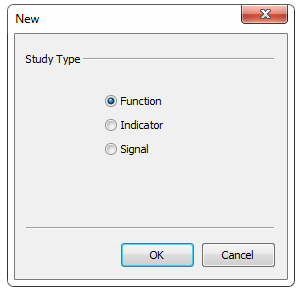In the next window, we have to give our new function a name. Following the naming from earlier lessons, I will call the function “ABC_MovingAverage_Lesson6”. I am using underscores within the name, as Function names may not contain any spaces and non-alphanumeric characters. The underscore is the only exception, that’s why I am using it instead of the space. Please feel free to give your function a different name. Just keep in mind that you will have to slightly adjust your code later.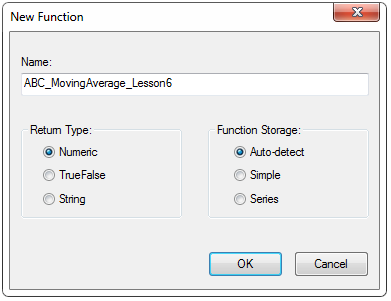The “Return Type” specifies the type of result that the function returns to the caller. For the moving average we will return a numeric value. So we set it to “Numeric”.

In case your function returns a boolean result, the return type should be TrueFalse. Finally you would use the last option, in case the function returns a string.

Set the “Function Storage” to Auto-detect and have the PL Editor take care of the correct storage. In general a function would be “Simple”, unless you reference previous bar’s values in it (using square brackets) – in that case it would become “Series”.

### Function Inputs

Our idea is to turn the moving average code from lesson 2 into a function. We also want to be able to easily call it with different length or using a different price for the average later.

Like the indicator, the function will have two inputs. These will allow you to set the length and price for the average. We will create similar inputs in the code that calls the function later. This way we can alter the results the function returns.

You have to specify a default input value in an indicator. In the function code on the other hand, you have to specify the type of each input. This will make sure that the program knows what input value to expect while the code is running.

Most of the time you will work with three types of function input parameters – Numeric, TrueFalse or String (text). Each parameter can have one of two main subtypes – series and simple. Although this might sound a bit more complex, it’s very easy when you keep the following in mind. If the particular input parameter is constant it’s “simple”. A moving average length for example would be “NumericSimple”. Inputs that refer to values that can change are of the subtype series. That’s why a price series input (like “Close” for the moving average) would be “NumericSeries”.

The rule of thumb is, when the input stays constant bar by bar it’s “Simple”, otherwise it’s “Series”.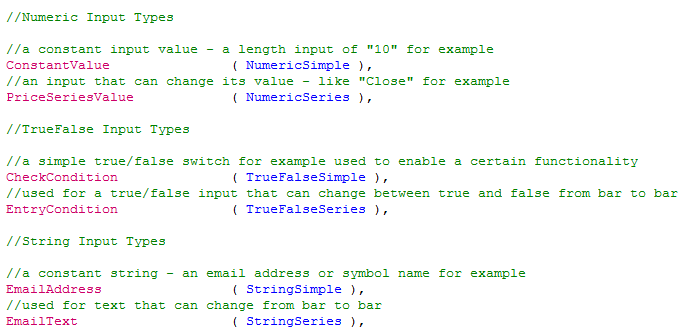### Function Outputs

You can also use inputs to receive values out of functions. These “outputs” are treated as inputs in the code as well. With these inputs you can reference values to the caller (indicator, signal or function). This way you can create a function that returns all values of many related calculations. The Stochastic function comes to mind as example here. It’s not just returning one, but four values (additionally to the normal function return value): Slow %K, Slow %D, Fast %K and Fast %D.

You declare a reference input similar to a regular input, the only difference is that you have to add “Ref” to the type. Most build in functions in Multicharts and Tradestation that are using reference inputs use the letter “o” in front of the input name. This way you can immediately see that an input/output (hence the “o”) is referencing values to the caller. I would suggest to keep this habit, since it can help making your code clearer and better to read.
The input parameter types are numeric, true/false, and string. That’s why the reference inputs are declared as numericref, truefalseref and stringref.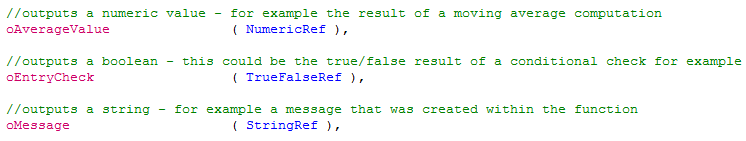### Return Value

These output values are in addition to the normal function return value. The latter is of the type specified as return type when you created the function. The normal return value is assigned to the function name in the code.

All Tradestation functions have to have a return value assigned to the function name. While Multicharts on the other hand doesn’t require that. You should make it your habit, as good practice, to always add one (even if it’s just a dummy value like +1), though. This dummy value can even be used later to check for a successful function call.

You can also pass and reference arrays to and from functions. This is done with NumericArray, NumericArrayRef, TrueFalseArray, TrueFalseArrayRef, StringArray or StringArrayRef. We will look at this topic in another lesson. For today, just keep in mind that you can pass and reference arrays, too.

### What parts do we need?

We want our function to compute a moving average and return the result. This is done by assigning the value to the function name.

From looking at the code we created in lesson 02, we will require two inputs:

• One for the price that should be averaged. From what we learned above, this will be a “NumericSeries” type input.
• An input for the length. As this will remain constant for the moving average, it will be of type “NumericSimple”.

Let’s use the same names for the inputs as we did in the lesson 02 indicator.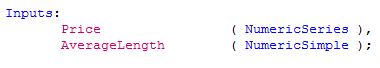### Function Code

The main function code will be very similar to the indicator, too. Our function requires a variable used as counter for the loop. Another variable to hold the result of the summation and finally a variable to hold the result of the division (the actual average result). While it would be possible to use the same variable for the two results, the code becomes more readable with using two.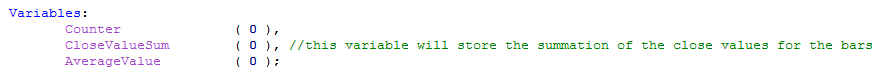We can also reuse most of the main code we wrote for the moving average indicator. It uses the “for loop” to sum the price values over the last X bars (where X is the AverageLength input value). To get the actual average result, we have to divide the result of the summation by the AverageLength.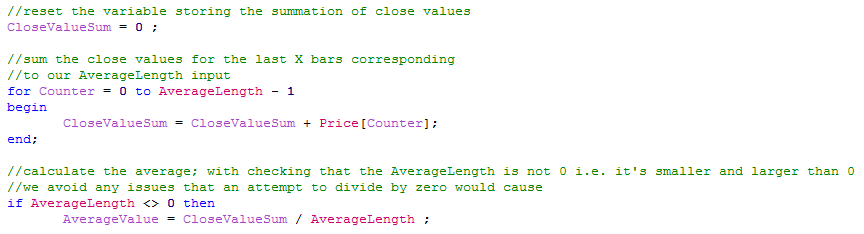As you can see there are only a few differences in the main code block. We can’t use the plot reserved word in a function and we need to assign the variable AverageValue to the function name instead. This way it can be returned to the code calling the function.Now click compile in the editor (or press F3) and you have created your first function. Good job.

### How do we call a function?

We should put our function to use as the next step and check if it in fact does what we want. The easiest way to do that is to call the function in an indicator and plot the value it returns. This way we can easily compare it to the indicator code we created in lesson 02.

To be able to easily customize the indicator on the chart, we want to be able to set the price used in the moving average and the length via inputs.

As the “heavy lifting” is done within the function code, our indicator just requires one variable that will store the function’s return value.

To receive the result, you simply assign the function name to the variable that you want to use. The function name is followed by an opening bracket and the first function input. If the function has more than one input, each input is separated by a comma. After the last input you close the bracket and end the code statement with a semicolon.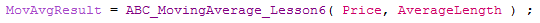In case you have a function without any inputs, the bracket would remain empty.

The full indicator with the function call could look something like this.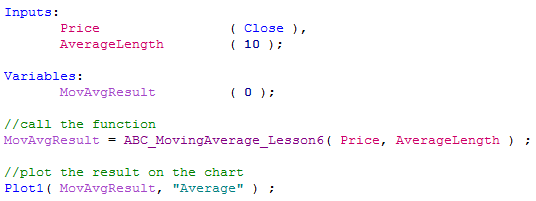### Comparing Results

Let’s compare this study to the one we created in lesson 02 and check if the results match. Provided we are using the same inputs and settings, they should – unless we made a mistake in our coding.

The function driven indicator is displayed on the chart as green line. The red line underneath it on the other hand is showing the result of the code we created in lesson two.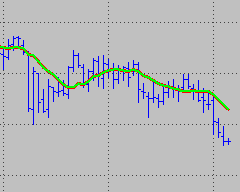We have an exact match and now have a function that is able to compute a simple moving average.

I would suggest to practice a little bit more how to call and handle functions. You can for example expand the indicator calling our function, so it is able to plot two different moving averages. The study should be able to display two averages, same length, but different prices. Or depending on the inputs, same prices, but different length.

### Conclusion

The lesson today might have made it a bit clearer how a function can help making your programming life easier. You can write code that is better to read and easier to maintain with them. Another benefit of functions is, you don’t necessarily have to know how the code works within it in order to use it. All you really need to know is how to call the function and how to handle the value or values it returns.

This concludes the lesson for today. We will come back to functions when we take a look at how to use the value area function that is part of our free Value Area Study.